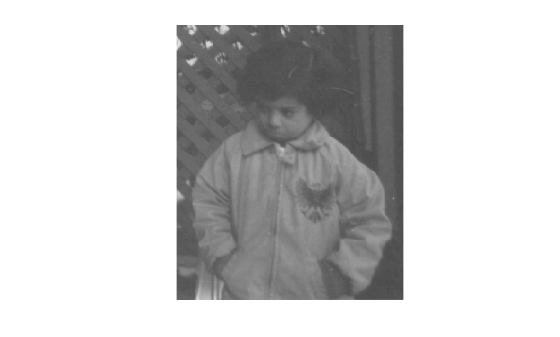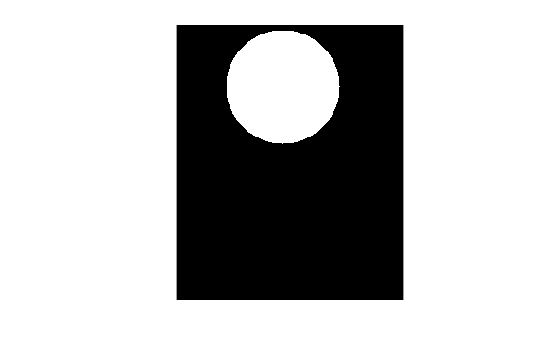# Create Binary Mask Using an ROI Function

This example shows to create a binary mask using one of the ROI creation functions, such as `drawcircle`, with the mask creation function `createMask`.

Read an image into the workspace and display it.

```img = imread('pout.tif'); h_im = imshow(img);```Create an ROI on the image using one of the ROI creation functions.

`circ = drawcircle('Center',[113,66],'Radius',60);`Create a binary mask from the ROI using `createMask`. The `createMask` function returns a binary image the same size as the input image. The pixels inside the ROI are set to 1 and the pixel values everywhere else are set to 0.

```BW = createMask(circ); imshow(BW)```